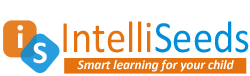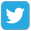Prepare forOlympiad Redeem Vouchers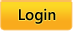Common Core:  MATH |  LANGUAGE ARTS |  SCIENCE
 Grades: Kindergarten |  First |  Second |  Third |  Fourth |  Fifth

# Common Core

Skills available for Common Core kindergarten math standards

Click on the name of a skill to practice that skill.

### Counting and Cardinality

Know number names and the count sequence.
• CC.K.CC.1 Count to 100 by ones and by tens.

• CC.K.CC.2 Count forward beginning from a given number within the known sequence (instead of having to begin at 1).

• CC.K.CC.3 Write numbers from 0 to 20. Represent a number of objects with a written numeral 0-20 (with 0 representing a count of no objects).

Count to tell the number of objects.
• CC.K.CC.4 Understand the relationship between numbers and quantities; connect counting to cardinality.

• CC.K.CC.4a When counting objects, say the number names in the standard order, pairing each object with one and only one number name and each number name with one and only one object.

• CC.K.CC.4b Understand that the last number name said tells the number of objects counted. The number of objects is the same regardless of their arrangement or the order in which they were counted.

• CC.K.CC.4c Understand that each successive number name refers to a quantity that is one larger.

Count to tell the number of objects.
• CC.K.CC.5 Count to answer “how many?” questions about as many as 20 things arranged in a line, a rectangular array, or a circle, or as many as 10 things in a scattered configuration; given a number from 1-20, count out that many objects.

Compare numbers
• CC.K.CC.6 Identify whether the number of objects in one group is greater than, less than, or equal to the number of objects in another group, e.g., by using matching and counting strategies. (Include groups with up to ten objects.)

• CC.K.CC.7 Compare two numbers between 1 and 10 presented as written numerals.

### Operations and Algebraic Thinking

Understand addition as putting together and adding to, and understand subtraction as taking apart and taking from.
• CC.K.OA.1 Represent addition and subtraction with objects, fingers, mental images, drawings (drawings need not show details, but should show the mathematics in the problem), sounds (e.g., claps), acting out situations, verbal explanations, expressions, or equations.

• CC.K.OA.2 Solve addition and subtraction word problems, and add and subtract within 10, e.g., by using objects or drawings to represent the problem.

• CC.K.OA.3 Decompose numbers less than or equal to 10 into pairs in more than one way, e.g., by using objects or drawings, and record each decomposition by a drawing or equation (e.g., 5 = 2 + 3 and 5 = 4 + 1).
• CC.K.OA.4 For any number from 1 to 9, find the number that makes 10 when added to the given number, e.g., by using objects or drawings, and record the answer with a drawing or equation.
• CC.K.OA.5 Understand addition as putting together and adding to, and understand subtraction as taking apart and taking from. Fluently add and subtract within 5.

### Number and Operations in Base Ten

Work with numbers 11-19 to gain foundations for place value
• CC.K.NBT.1 Compose and decompose numbers from 11 to 19 into ten ones and some further ones, e.g., by using objects or drawings, and record each composition or decomposition by a drawing or equation (such as 18 = 10 + 8); understand that these numbers are composed of ten ones and one, two, three, four, five, six, seven, eight, or nine ones.

### Measurement and Data

Describe and compare measurable attributes.
• CC.K.MD.1 Describe measurable attributes of objects, such as length or weight. Describe several measurable attributes of a single object.

• CC.K.MD.2 Directly compare two objects with a measurable attribute in common, to see which object has “more of”/“less of” the attribute, and describe the difference. For example, directly compare the heights of two children and describe one child as taller/shorter.

Classify objects and count the number of objects in each category.
• CC.K.MD.3 Describe measurable attributes of objects, such as length or weight. Describe several measurable attributes of a single object.

• CC.K.MD.4 Classify objects into given categories; count the numbers of objects in each category and sort the categories by count. (Limit category counts to be less than or equal to 10.)

### Geometry

Identify and describe shapes (squares, circles, triangles, rectangles, hexagons, cubes, cones, cylinders, and spheres).
• CC.K.G.1 Describe objects in the environment using names of shapes, and describe the relative positions of these objects using terms such as above, below, beside, in front of, behind, and next to.

• CC.K.G.2 Correctly name shapes regardless of their orientations or overall size.

• CC.K.G.3 Identify shapes as two-dimensional (lying in a plane, “flat”) or three-dimensional (“solid”).

Analyze, compare, create, and compose shapes
• CC.K.G.4 Analyze and compare two- and three-dimensional shapes, in different sizes and orientations, using informal language to describe their similarities, differences, parts (e.g., number of sides and vertices/“corners”) and other attributes (e.g., having sides of equal length).
• CC.K.G.5 Model shapes in the world by building shapes from components (e.g., sticks and clay balls) and drawing shapes.

 Connect and Follow About Intelliseeds Learning My Account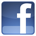Facebook Benefits Membership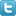Twitter Plans and Pricing Skills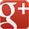Google+ FAQ Wall of Fame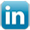LinkedIn Newsletter Student Reports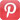Pinterest Careers Rewards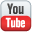Youtube Contact Us What is IntelliAbility?Our Partners Terms & Conditions Tell a FriendNon Profit Partners Testimonials User Guide PTA Fundraising Offers Common Core Math Schools Common Core ELA Virginia SOL Content Team
 Welcome to Intelliseeds Learning Smart learning for your child Intelliseeds learning provides practice in several subjects including Math, Reading Comprehension, Language Arts, Logical Reasoning, Mental Ability, Analytical Ability and Critical Thinking, which are essential to the success of every student. Skills are organized by grades, topics, practice tests and time bound tests. The Math section covers skills such as counting, number operations, algebra, story problems and many more. The IntelliAbility section focuses on thinking skills, patterns, sequences, problem analysis, analogies and over 50 other topics to help develop your child's brain. Click here to learn more about IntelliAbility. The Language Arts section includes reading comprehension, nouns, verbs, adverbs, adjectives, predicates, vowel sounds and many more grammar skills.Privacy | © 2023 Intelliseeds Learning™